## Artificial life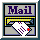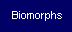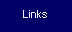LogiCell 1.0

Since the Java Plugin is now outdated, you can :

Or use Java Web Start

 Download that text in PDF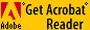Logicell
Quickstart
Functioning of Logicell
A- Short reminders about Boole's algebra
B- Automatism and booleans functions
C- Basic principles

D-Construction of Boolean operators (Gates)
E- Construction of an equation
F- Construction of a program

Introduction to Cellular Automata

 LogiCell is presented in "Collision-Based Computing", Adamatzky A. (ed.), Springer, 2002. This book is the last reference in that field. You can download a PDF version of that text there: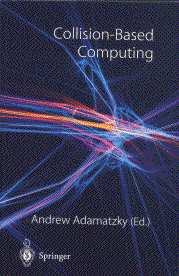Une ligne juste pour maintenir la largeur de la cellule sous netscape. Une ligne juste pour maintenir la largeur de la cellule sous netscape.

LogiCell

We have seen in Introduction to CA that the Game of Life has Universal computation capabilities. LogiCell shows how the use of guns as generators, gliders as signals and eaters as outputs, can ensure the computation of any Boolean functions.

The applet theoretically permits to test any four input Boolean equation (they may be indefinitely repeated).

A set of combinatory applications shows how to use these functions.

One note concerning speed. The response times indicated is that of my P400 with IE5.0.

Quickstart

When starting, a working mode must be selected, i.e. :

1. Equation : in this mode, you can test a Boolean equation, theoretically without limitation. To enter a new equation, select the key " New " and set the equation with the different input buttons. Its possible to validate only if the input is syntactically complete. Then fix the values of ABCD Boolean entries and launch the algorithm. A black output means false, a white one true.
2. Two-way switch : youve got a switch at each ends of your lane, if you change one of both switches, it changes the state of the lamp. Its the only dynamic mode, you neednt validate the input, the algorithm is automatically launched. The equation is ~(A w B).
3. Binary adder : Its a calculator that administers the sum of two one bit numbers (I admit it can hardly be more limited). The two input values are A (false = 0, true = 1) and B. The result is naturally expressed in binary and expresses itself over two bits. For example if A and B are true, the sum of A + B is 1+1 = 2 i.e. 10b in binary notation.
4. Digit : basic liquid crystal screens display the different characters on the basis of a seven segments matrix. If a binary number is expressed from the four binary inputs DCBA (A being the light weighted bit, DCBA = 0111, is worth 7 for example), the switching on of each segment can be managed by a Boolean function. Choose a number  the decimal value appears in the text zone  and watch the different segments light. In this first mode, three segments over seven are given so as to fasten the treatment.
5. Two bits binary adder : its the same as the former one, but it can add two numbers expressed over two bits DC + BA. For example, with D : true, C : false, B : false and A : true, weve got the sum 10+01, i.e. in decimal : 2+1, the binary result is 11, i.e. 3. The decimal values are shown in the text zone.
6. Digit 2 : its the same as the former one, but this time it manages the whole 7 segments. Be patient, or better join my site while the algorithm is working.

For the rest, the interface is simple enough to be spontaneously understood.

Functioning of LogiCell

A- Short reminders about Boole's Algebra1

Booles algebra, (that is in fact a set of algebra structures) was introduced in 1847 so as to propose an algebraic formulation of logical proposals. This algebraic formulation is made necessary by the ambiguity and complexity of the proposals when expressed through words. Lets consider, for example, the following proposals :

- If at night, all cats are gray and if Garfield is a cat, then Garfield is gray at night.

We recognise a syllogism, form of expression studied since the fifth century before JC as an instrument of codification of a speech and generalised by Aristotle. This proposal is obvious (at least logically)

- If a cat ate the mouse and if Garfield is a cat, the Garfield ate the mouse.

This second proposal is obviously false, but the error doesnt appear spontaneously and its necessary to reason about it (in this case, Garfield is not the only existing cat  and he is all the more the less likely cat to perpetrate such a crime) to become conscious of the error. This example is a trivial one, but imagine a complex set of linked proposals, it then quickly becomes impossible to jump to the validity of the conclusion.

Boole's algebra permits to solve this type of problems.

This algebra works on two values : 1 and 0 (true and false) and uses three operators :

1. The conjunction ( noted ^) i.e. the logical AND ;
2. The disjunction : (noted v) i.e. the logical OR ;
3. The negation (noted ~) i.e. the logical NO.

The properties of these operators can be expressed in a truth table

Logical AND (^)
 p q p ^ q 1 1 1 1 0 0 0 1 0 0 0 0

Here, a proposal is true only if both premises are true : " if the weather is fine and if there is snow, Ill go skiing " if one of these two conditions is not fulfilled, the proposal " Ill go skiing " is false.

Logical OR (v)
 p q p v q 1 1 1 1 0 1 0 1 1 0 0 0

Here, if one of the premises is true, the conclusion will be true too " if my alarm clock doesnt ring or if my car refuses to start, then Ill be late at work.".

Logical NOT (~]
 p ~p 1 0 0 1

This operator simply serves to invert the value of a proposal.

Another operator frequently used (notably in LogiCell) is the exclusive OR (Xor) noted w :

XOR (w)
 p q pwq 1 1 0 1 0 1 0 1 1 0 0 0

In opposition to the classical OR, the conjunct validity of two proposals induced a false conclusion. This operator is not an elementary one, it can be built from the former three ones, for example p w q = (p^~q)v(~p^q). Generally, all operators ( implication, equivalence) can be elaborated from these three elementary operators.

Operators composition laws then ensure Boole's algebra to manage rigorously a complex set of linked proposals.

This algebra is massively used as well in mathematics (demonstration theory, probabilities) as for more practical things (automatism, computing). As far as were concerned its interest comes from the fact that the three Boolean operators are theoretically necessary and sufficient to build a Universal Turing Machine2.

B- Automatism and Boole's algebra

To show the practical use of Boolean functions, we chose to present a few automatism applications.

An automatism is a system placed between an operator and a machine. The operator determines the inputs of the automatism in function of the desired result, and the automatism realises a set of operations that determine the values of the outputs sent to the machine. The searches made about automation problems led to the elaboration of a set of mathematical tools (" automatic ") whose use widely overflowed the original field.

Lets take the easy example of a double command. Imagine a stamping press. Its a machine that (under high pressure) activates a die that will distort a piece of metal to give it a special form. An aspirin tube, for example, is made by the distortion of a piece of metal roughly looking like a coin. To make sure that the hands of the operator are not in the die zone he has to action two switches simultaneously, one for each hand. The electric scheme is an elementary one :

A double command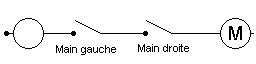Both switches must be locked if you want the electricity to each the engine (or, in this case, the relay that will initiate the hydraulic jack). Two conditions must be simultaneously met : this is the Boolean operator AND. If the two switches are plugged in parallel (and not in series as before) youll get the function OR. One switch closed will be enough to activate the output.

The association of Boolean functions then permits, by a complex treatment of inputs, to build programs - algorithms - reckoning the desired outputs.

The output of a double command exclusively depends on the state of inputs at the "i instant". In fact, the industrial automata also have memory functions. This is named combinatory applications. In fact it is often necessary to determine the output not only in function of the inputs at the "i instant", but also of the state of the inputs at the "i-1 instant". These are sequential applications3. LogiCell doesnt have any memory function, it is then limited to combinatory applications. The following examples will anyway show these ones can also be complex.

C- Basic principles

LogiCell is a pure Conway. A p30 gun in association with an eater generates the inputs.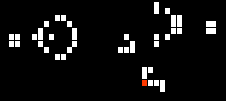The input is on the red cell. If it is on true (or 1), the eater will disappear and let the glider spread. Otherwise the glider is obviously absorbed.

An eater manages the output. The red displayed cell only is activated if an eater absorbs a glider. This cell is the output.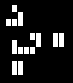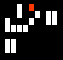Finally the simple guns (i.e. without eater) are used to build logic operators. Their interest comes from the ability of gliders to destroy each others. In LogiCell, the process takes two steps, the first meeting produces two blocks (2x2) then these ones are destroyed by the two following gliders. The introduction of a vertical offset of one cell would have avoided the phase of blocks. The periodicity of the gun allowed me to put the gliders at the same level to simplify the construction of operators.

The only links between the applet and the automata then are :

1. The position of the input cell. There is naturally a gun and then an input cell by input of the equation (ABCD) eventually repeated.
2. The position of the output cell. There is a unique output for each equation.

The automata deals alone with inputs and outputs, it solves the problem on its own.

D- Construction of Boolean operators

The three logical operators AND, OR, NO, manage alone the whole Boolean operators (NotAnd or NotOr could have been used too). They are built that way :

And gate with B true and A falseThe operator is constructed with two inputs and an opposite direction gun. The output is on the trajectory of the glider of input B. the configurations are :

1. Both inputs are false : right gun blocks the B glider.
2. A is true and B is false : A blocks the right gun. B is false and therefore can't join the output.
3. A is false and B is true : The right gun blocks B which can't join the output.
4. A and B are true : A blocks the right gun, B can join the output.

Gate Or with A and B trueThe operator is constructed with two inputs, a same direction gun and an opposite gun. The output is on the trajectory of the glider of input B. The configurations are :

1. Both entries are false : the leftmost gun is blocked by the right gun.
2. A is true and B is false : A blocks the right gun, the left gun can't join the output.
3. A is false and B is true : B blocks the right gun, the left gun can't join the output.
4. A and B are true : A blocks the right gun, B is stopped by the eater, the left gun's glider can join the output.

Gate Not with A true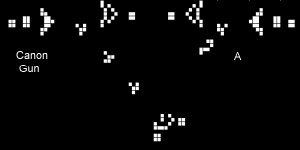Not A (~A) sends back the opposite of A. The output is on the trajectory of the left gun. The configurations are :

1. A is true : the glider of A blocks the gun that then cant join the output ; the result is false.
2. A is false : the gun propagates freely towards the output ; the result is true.

We note that gate A inverts the direction of the output. If an input uses a glider sliding towards the right, the output is on a left glider and vice-versa.

The other logic operators can be built from the three former ones. For example, LogiCell builds the operator Xor as :

A Xor B = (A Or B) And Not(A And B)
AwB = (AvB) ^ ~(A^B)

E- Construction of an equation

An equation is built from the association of several operators. Each operator having two inputs and one output, we use the output of the first operator as an input for the following one. For example :

Equation A And B And C (A^B^C)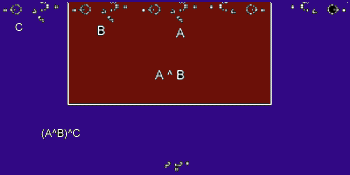The red zone shows a classical AND operator without its output eater. The output of this gate, here Bs glider, is associated to the input C to build the equation (A^B)^C, the output eater is then Cs glider.

This mechanism permits to generate the whole possible combinations, and then the matching equations. The algorithm of LogiCell can manage any number of inputs, the program is limited to four distinct inputs only for simplicity and interface.

F- Construction of programs

Automatism components (algorithms) manage multiple outputs. LogiCell associates various equations on one grid. The program then manages the different outputs according to the problem. We note here the parallel treatment of different equations.

LogiCell proposes some combinatory applications as illustration :

1. Two-way switch : its the classical system of inversion of the state of a bulb managed by two switches : you switch on your corridor when going out of the living room, cross it, and switch it off when you reach the door. When you come back, you do exactly the opposite. In this case, we have two entries (both switches) and one output. The equation is : Not (A Xor B), ~(AwB).
2. One bit binary adder : weve got two input bits (A and B) that are added. The result is expressed over two bits : one bit of sum and one of carry over. For those who wish they would understand the equations, I suggest a visit of Patrick Traus site at http://www-ipst.u-strasbg.fr/pat/autom/.
1. Sum : A Xor B ; AwB.
2. Carry over : A And B ; A^B.
3. Digit : former liquid crystal screens displayed numbers through a seven-segments matrix.

 7 segments display. The number 5.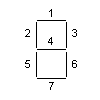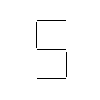The value to display is built in binary from the four input bits under the form DCBA, A being the light bit. For example the configuration D false, C true, B false A true is worth 0101 binary or 5 decimal. To display the number 5, the segments 12467 must be lit. Each segment is managed by an equation :

1. Segment 1: Not (A Xor C) Or B Or D ; ~(AwB)vCvD.
2. Segment 2: (C And Not D And Not(A And B)) Or D Or (Not A And Not B And Not C And Not D) ; (C^~D^~(A^B))vDv(~A^~B^~C^~D).
3. Segment 3: Not(A Xor B) Or Not C ; ~(AwB)v~C.
4. Segment 4: (B And C And Not A) Or (B Xor C) Or D ; (B^C^~A)v(BwC)vD.
5. Segment 5: (Not C Or B) And Not A ; (~CvB)^~A.
6. Segment 6: A Or C Or Not B Or D ; AvCv~BvD.
7. Segment 7: (Not A Or B Or C Or D) And (A Or B Or Not C Or D) And (Not A Or Not B Or Not C Or D) ; (~AvBvCvD)^(AvBv~CvD)^(~Av~Bv~CvD).

The equations for 2, 4 and 7 are particularly long. To accelerate the result, in the first display mode, these segments are managed by the program, the automata only manages the four other segments. The mode "Digit 2" manages the seven segments.
4. Two bits binary adder : the principle is the same as for the former one, but we manage 2 bit numbers. We then add BA+DC, and the result is over 3 bits. For example B true and A false give 10 binary, i.e. 2 decimal, D false and C true give 01 binary, i.e. 1 decimal. The sum 10+01 binary (2+1 decimal) is worth 101 binary (3 decimal). The 3 output bits are :
1. The sum of both low weights bits A+C :
A Xor C ; AwC.
2. The sum of B and D, plus the eventual carry over of A+C :
DwBw(A^C).
3. The carry over of the former sum :
(B^D)v((BwD)^(A^C))..

LogiCell is unquestionably technical; its contribution to artificial life is not a direct one. It nevertheless shows an essential part of what we mean when it is said that a Conway automaton, a Life Game, is a Turing machine.

2- Heudin JC, L'évolution au bord du chaos, Hermès, Paris, 1998, p. 122. NAND (Not-And) or NOR (Not-Or) can also generate every Boolean functions.

3- Patrick Trau, IPST, Université Louis Pasteur Strasbourg. http://www-ipst.u-strasbg.fr/pat/autom/.

Jean-Philippe Rennard, Ph.D., 12/2000.
http://www.rennard.org/alife
alife@rennard.org

Copyright : This text has been written for an educational purpose. It is free for any private use. If you want to use it for a non-commercial public purpose, please quote author and source. Commercial use is strictly forbidden unless written agreement.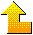Home - Cellular Automata - Biomorphs - Genetic Algorithms - Ants - Links